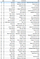# How To Web Scrape

Using Python’s Beautiful Soup

# Step 1

`import requestsfrom requests import getfrom bs4 import BeautifulSoupimport pandas as pdimport numpy as npimport pandas as pd`

# Step 2

`url = 'https://en.wikipedia.org/wiki/The_Bachelorette_(season_16)'results = requests.get(url)`

Then run results alone to check the response.

`results`

The response should look like this <Response >. If the response is not 200 that means there is an error.

From the url we’ll be scraping some columns from the following table.

# Step 3

`soup = BeautifulSoup(results.text, "html.parser")print(soup.prettify())`

# Step 4

After hitting “Inspect” you will see the following.

# Step 7

`soup.findAll('table', class_='wikitable')`

# Step 8

`soup = soup.findAll('table', class_='wikitable')`

Next

`soup = soup.find('tbody')soup`

# Step 9

`x = soup.find_all('tr')x`

# Step 10

`x.find_all('td').find('a')['title']`

# Step 11

`soup.find_all('tr').find_all('td').find('b').text`

# Step 13

`soup.find_all('tr').find_all('td').text.replace('\n','')`

# Step 14

`names = []for n in range(3,36):    names.append(soup.find_all('tr')[n].find_all('td').text.replace('\n',''))names`

To get rid of the extra characters, the brackets and the numbers, another for loop was created.

`for i in range(len(names)):    num = names[i].index('[')    names[i] = names[i][:num]names`

# Step 15

`hometown = []for x in range(3,36):    hometown.append(soup.find_all('tr')[x].find_all('td').text.replace('\n',''))    jobs = []for n in range(3,36):    jobs.append(soup.find_all('tr')[n].find_all('td').get_text().replace('\n',''))ages = []for age in range(3,36):    ages.append(soup.find_all('tr')[age].find_all('td').text.replace('\n',''))list(map(lambda x: int(x), ages))`

# Step 16

`import pandas as pdfrom pandas import DataFramedf = pd.DataFrame(ages, columns=['ages'])df['names'] = namesdf['hometowns'] = hometowndf['jobs'] = jobsdf`

# Step 17

`new_row1 = {'ages': [31, 36], 'names':['Dale Moss', 'Zac Clark'], 'hometowns': ['Brandon, South Dakota', 'Haddonfield, New Jersey'], 'jobs': ['Former Pro Football Wide Receiver', 'Addiction Specialist']}top_row = pd.DataFrame(new_row1)df = pd.concat([top_row, df]).reset_index(drop = True)df`All names included

## More from Raizel Bernstein

Aspiring Data Scientist